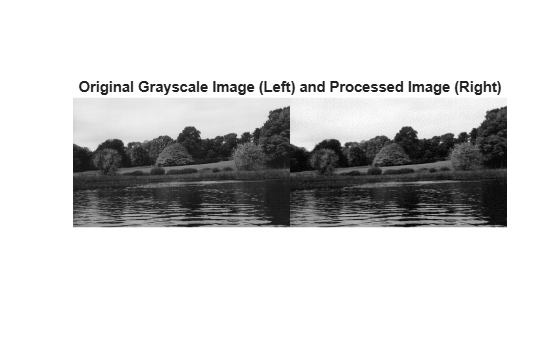# dct2

2-D discrete cosine transform

## Syntax

``B = dct2(A)``
``B = dct2(A,m,n)``
``B = dct2(A,[m n])``

## Description

example

````B = dct2(A)` returns the two-dimensional discrete cosine transform of `A`. The matrix `B` contains the discrete cosine transform coefficients B(k1,k2).```
````B = dct2(A,m,n)` and`B = dct2(A,[m n])` pad the matrix `A` with `0`s to size `m`-by-`n` before applying the transformation. If `m` or `n` is smaller than the corresponding dimension of `A`, then `dct2` crops `A` before the transformation.```

## Examples

collapse all

This example shows how to remove high frequencies from an image using the two-dimensional discrete cosine transfer (DCT).

Read an image into the workspace, then convert the image to grayscale.

```RGB = imread('autumn.tif'); I = rgb2gray(RGB);```

Perform a 2-D DCT of the grayscale image using the `dct2` function.

`J = dct2(I);`

Display the transformed image using a logarithmic scale. Notice that most of the energy is in the upper left corner.

```figure imshow(log(abs(J)),[]) colormap(gca,jet(64)) colorbar```Set values less than magnitude 10 in the DCT matrix to zero.

`J(abs(J) < 10) = 0;`

Reconstruct the image using the inverse DCT function `idct2`.

`K = idct2(J);`

Display the original grayscale image alongside the processed image.

```figure imshowpair(I,K,'montage') title('Original Grayscale Image (Left) and Processed Image (Right)');```## Input Arguments

collapse all

Input matrix, specified as a 2-D numeric matrix.

Number of image rows, specified as a positive integer. `dct2` pads image `A` with `0`s or truncates image `A` so that it has `m` rows. By default, `m` is equal to `size(A,1)`.

Number of image columns, specified as a positive integer. `dct2` pads image `A` with `0`s or truncates image `A` so that it has `n` columns. By default, `n` is equal to `size(A,2)`

## Output Arguments

collapse all

Transformed matrix using a two-dimensional discrete cosine transform, returned as an `m`-by-`n` numeric matrix.

Data Types: `double`

collapse all

### Discrete Cosine Transform

The discrete cosine transform (DCT) is closely related to the discrete Fourier transform. It is a separable linear transformation; that is, the two-dimensional transform is equivalent to a one-dimensional DCT performed along a single dimension followed by a one-dimensional DCT in the other dimension. The definition of the two-dimensional DCT for an input image `A` and output image `B` is

where

and

M and N are the row and column size of `A`, respectively.

## Tips

• If you apply the DCT to real data, the result is also real. The DCT tends to concentrate information, making it useful for image compression applications.

• To invert the DCT transformation, use `idct2`.

 Jain, Anil K., Fundamentals of Digital Image Processing, Englewood Cliffs, NJ, Prentice Hall, 1989, pp. 150–153.

 Pennebaker, William B., and Joan L. Mitchell, JPEG: Still Image Data Compression Standard, Van Nostrand Reinhold, 1993.